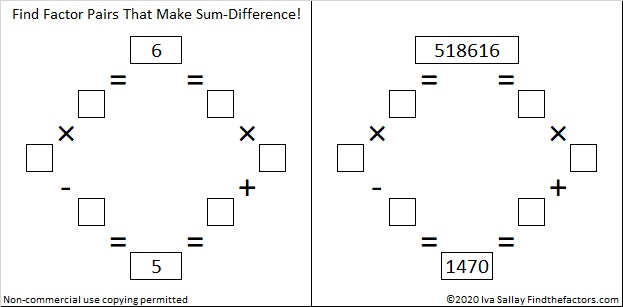# 1470 Can You Find Factor Pairs That Make Sum-Difference?

Contents

### Today’s Puzzles:

I bet you can find a factor pair of 30 that adds up to 13 as well as another factor pair of 30 that subtracts to give you 13.

If you can solve that simple puzzle, then you will be able to solve the puzzle next to it. Even though 1470 has 12 different factor pairs, you don’t have to worry too much about them: All of the answers to the second puzzle are just _____ times the answers to the first puzzle! (And 1470 is _____² times 30.)Likewise, don’t get scared off with this next set of puzzles one of which wants you to find the factor pairs of 518616 that add up or subtract to 1470. Crazy, right? Again, if you can solve the first puzzle in the set, and if you can multiply a 3-digit number by a 1-digit number, you can easily solve the second puzzle because the answers are just _________ times the answers to the first puzzle in the set! (And 518616 is merely _________² times 6.)If you need more help than what I’ve already said, scroll down to the factor trees for 1470. I selected those particular trees for a reason!

### Factors of 1470:

• 1470 is a composite number.
• Prime factorization: 1470 = 2 × 3 × 5 × 7 × 7, which can be written 1470 = 2 × 3 × 5 × 7²
• 1470 has at least one exponent greater than 1 in its prime factorization so √1470 can be simplified. Taking the factor pair from the factor pair table below with the largest square number factor, we get √1470 = (√49)(√30) = 7√30
• The exponents in the prime factorization are 1, 1, 1, and 2. Adding one to each exponent and multiplying we get (1 + 1)(1 + 1)(1 + 1)(2 + 1) = 2 × 2 × 2 × 3 = 24. Therefore 1470 has exactly 24 factors.
• The factors of 1470 are outlined with their factor pair partners in the graphic below.### A Few Factor Trees for 1470:### More Facts about the Number 1470:

1470 is the average of 14² and 14³. That simple fact makes 1470 the 14th Pentagonal Pyramidal Number.

1470 is the hypotenuse of a Pythagorean triple:
882-1176-1470 which is (3-4-5) times 294

Hmm… that same factor pair showed up again!

This site uses Akismet to reduce spam. Learn how your comment data is processed.